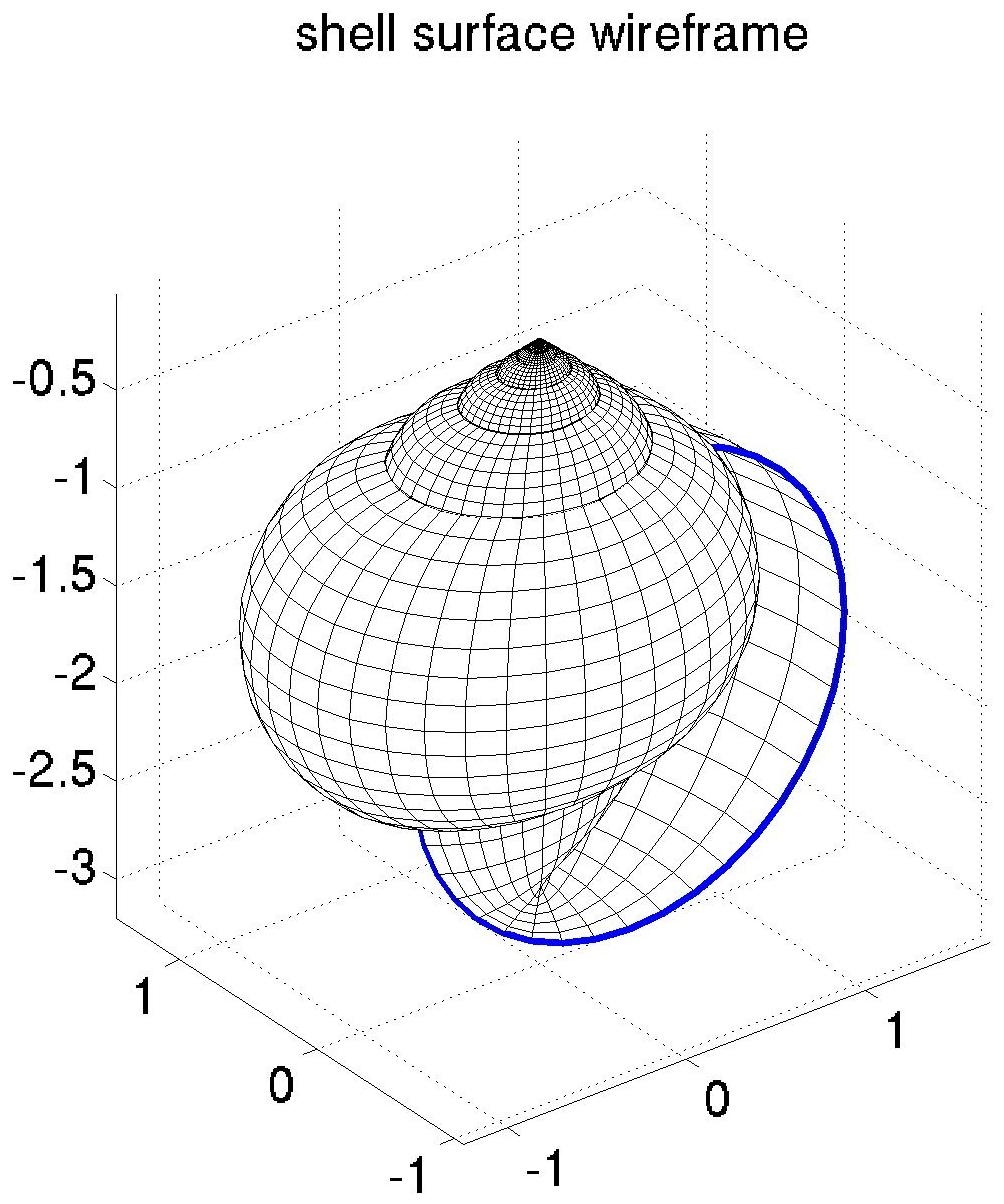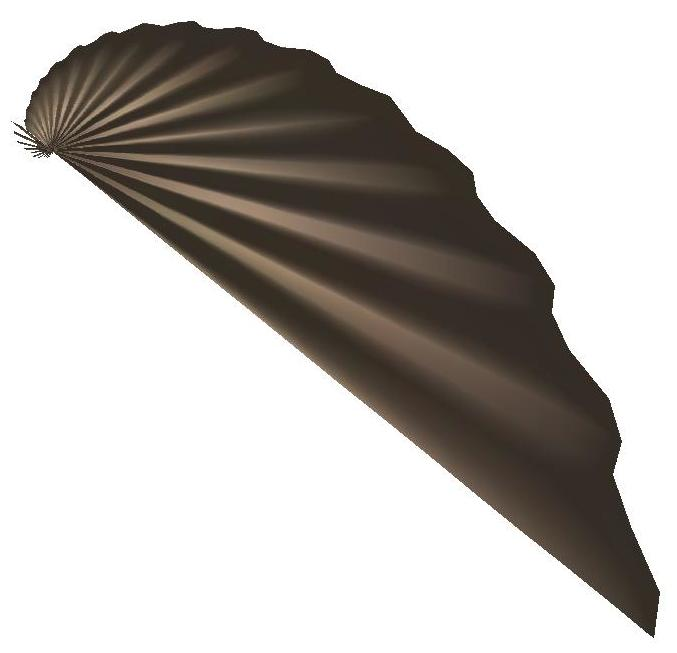# Sea Shells

There are a large variety of sea-shells (and land shells) that can be simply described by rotating a ellipse around a log-spiral that has been projected onto a cone. The image below was generated using this approach:

The following images show the log-spiral, the spiral on the cone, a circle rotated about the spiral, and the resulting surface.

They were generated with the matlab function shells.m, which is hopefully self-explanitory if you read the comments.

You can generate many other shell shapes with the same code. Some examples calling shells in Matlab are given below.

 clear a = 0.8; h = 2; alpha = -0.1; r1= 1.2; r2 = 1.2; shellsclear a = 1; h = 0; alpha = -0.1; r1 = 0.5; r2 = 1.0; shellsclear a = 1; h = 0; alpha = -1; r1 = 0.55; r2 = 1; N = 1/2; shellsclear a = 1; h = 0; alpha = -1; r1 = 0.55; r2 = 1; N = 1/2; f = 32*(1:2:15); A = 0.05*2.^[0:-2:-14]; N_phi = 120; shells